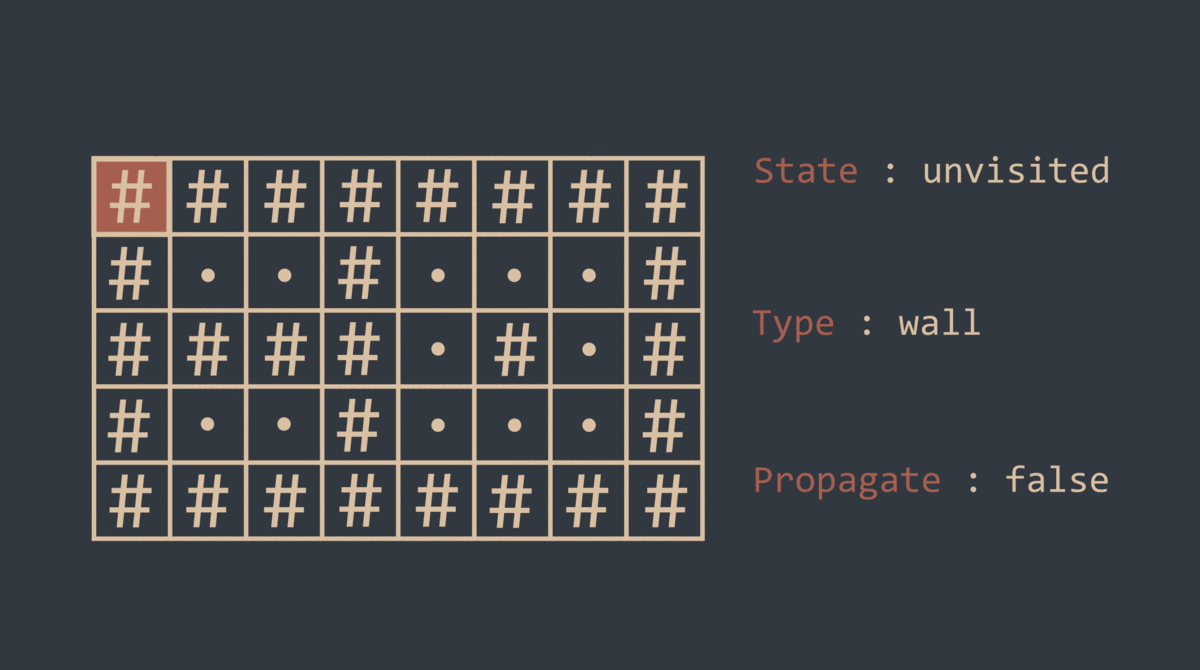# Counting Rooms Solution

14 December 2020

Statement

( The original statement can be found here )
You are given a map of a building, and your task is to count the number of its rooms. The size of the map is n×m squares, and each square is either floor or wall. You can walk left, right, up, and down through the floor squares.

Input
The first input line has two integers n and m: the height and width of the map. Then there are n lines of m characters describing the map. Each character is either . (floor) or # (wall).

Output
Print one integer: the number of rooms.

Constraints
1 ≤ n, m ≤ 1000
Time limit : 1.00s
Memory limit : 512MB

Example

Input :
5 8
# # # # # # # #
# .  .  # .  .  .  #
# # # # .  # .  #
# .  .  # .  .  .  #
# # # # # # # #

Output :
3

Solution

The problem asks us to calculate the number of rooms on the map, in other words, to calculate the number of groups consisting of connected dots. One way to solve this problem is to consider the given grid as a graph where the floor characters represent the nodes and the vertical/horizontal adjacencies represent the edges. In this way, we transform this problem into a classical "connected component counting" problem that can be solved with some kind of graph search algorithm, such as depth-first search , breadth-first search or even floodfill.

The following code shows a recursive depth-first search solution that uses a boolean two-dimensional array to keep track of the visited rooms. When the algorithm finds an unvisited room cell, it increments the answer by one and it propagates recursively through its neighbors and marks them as visited to avoid recounting the same room several times.

```#include <bits/stdc++.h>
using namespace std;

int neighborX = {0, 0, 1, -1};
int neighborY = {1, -1, 0, 0};

int n, m, answer = 0;
int vis;
char grid;

bool isValid (int y, int x) {
if (y < 0) return false;
if (x < 0) return false;
if (y >= n) return false;
if (x >= m) return false;
if (grid[y][x] == '#') return false;
return true;
}

void DFS (int y, int x) {
vis[y][x] = 1;
for (int i = 0 ; i < 4 ; i++) {
int newX = x + neighborX[i];
int newY = y + neighborY[i];
if (isValid(newY, newX)) {
if (!vis[newY][newX]) {
DFS(newY, newX);
}
}
}
}

int main() {
cin >> n >> m;
for (int i = 0 ; i < n ; i++) {
for (int j = 0 ; j < m ; j++) {
cin >> grid[i][j];
vis[i][j] = 0;
}
}
for (int i = 0 ; i < n ; i++) {
for (int j = 0 ; j < m ; j++) {
if (grid[i][j] == '.' && !vis[i][j]) {
DFS(i, j);